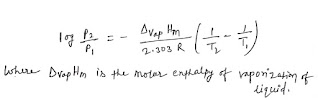# Liquid state state of matter  (Liquid  state)

## VAPOUR PRESSURE

The vapour pressure of a liquid may be defined as the pressure of the vapour in equilibrium with the liquid. The vapour pressure of a liquid increases with the increase in temperature. The governing expression isgoverning expression

The vapour pressure of a solvent invariably decreases when a nonvolatile solute is dissolved in it. This is due to the availability of lesser number of solvent molecules at its surface as compared to that available in pure solvent.

the boiling point of a liquid can be defined as the temperature at which is vapour pressure is equal to the external pressure. If the latter is equal to 1 atm, the corresponding temperature is known as the normal boiling point of the liquid.

## SURFACE TENSION

The surface tension of a liquid is defined as the force acting along the surface of a liquid at right angle to any line of unit length. Its Si unit is N m and is equal to 10³ dyn cm^-1 he surface tension (symbol: y) of a liquid decreases with increase ot temperature. The governing expression issurface tension
where M is the molar mass, v is the specific volume and Tc is the critical temperature

The surface active materials Such as soap, salts of higher sulphonic acids and higher amines lower the surface tension oi water. 1 he cleansing action of soap or detergent solution is due to the lowering of Surface tension between water and grease. The orientation or soap molecules at the surface is specific,  -CO0 groups pointing towards the Surface or water and hydrocarbon chains pointing
outwardly. These hydrocarbon chains act as a solvent for the grease and thus the latter is detached from the cloth along with the dust.

The capillary action (rise or fall of a liquid level within the capillary tube) is due to the surface tension of the liquid. The expression governing this phenomenon is given by

where y is surface tension, IS the radius of capillary tube, p is the dens liquid, g is acceleration due to gravity and 7 is the rise of liquid is Capillary tube.in a laboratory, the surface tension of a liquid can be determined by using a stalagmometer The liquid taken in the stalagmometer is allowed to fall drop-wise . The average weight of the liquid drop
is related to its surface tension by the expression

mg=(2πr)Y

Y= gama
where r is the radius of capillary tube of the stalagmometer. To avoid the measurement of r, the relative method is used where water(whose surface tension is known) is used as a reference liquid.

## Viscosity

The viscosity of a liquid is due to the forces attraction between molecules flowing in different layers in a laminar flow of the liquid. The viscosity (or more precisely, the coefficient of viscosity) may be defined as the force per unit area required to maintain velocity deterrence of unity between two par-
all layers of liquid unit distance apart. Its SI unit is Nm^-2 s. (=Pa s) and is equal to 10 dyn cm^-2 s(=10 poise). With increase in temperature, the viscosity of a liquid decreases due to decrease in the molecular attractions effect may be compared with the effect observed in gases where
increases with increase in temperature. In case of gases, viscosity
transfer of molecules (along with their momenta) from on is due to the transfer of molecules

The relation governing the variation of viscosity of a liquid with temperature is

ƞ= A exp(E/RT)

where A is a constant and E is the activation energy for The viscosity of a liquid
invariably increases with impurities. The associated liquids such as water, alcohols
and glycerol have higher viscosity as compared to the non associated liquids (benzene, toluene, etc)